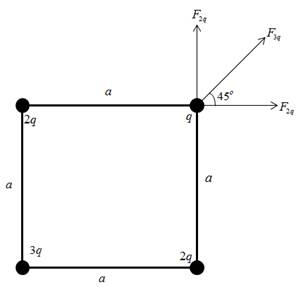Chapter 15, Problem 8P

Chapter
Section
Textbook Problem

Four point charges are at the corners of a square of side a as shown in Figure P15.8. Determine the magnitude and direction of the resultant electric force on q, with ke, q, and a left in symbolic form.To determine
The magnitude and direction of resultant electric force.

Solution:

The magnitude of resultant electric force is 4.33(q2kea2) .

The direction of resultant electric force is 45ο above the horizontal.

Explanation

The force diagram is given by,In the above diagram,

• F2q is the force due to the charge 2q on q.
• F3q is the force due to the charge 3q on q.

Formula to calculate the force due to the charge 2q on q is,

F2q=2q2kea2      (I)

• ke is the Coulomb constant.

Formula to calculate the force due to the charge 3q on q is,

F3q=3q2ke(2a)2       (II)

Net force along the x direction is,

Fx=F2q+F3qcos45ο       (III)

Net force along the y direction is,

Fy=F2q+F3qsin45ο       (IV)

Formula to calculate the magnitude of resultant electric force is,

FR=Fx2+Fy2       (V)

Substitute Equations (III) and (IV) in (V).

FR=(F2q+F3qcos45ο)2+(F2q+F3qsin45ο)2      (VI)

Substitute Equations (I) and (II) in (VI).

FR=(2q2kea2+3q2ke(2a)2cos45ο)2+(2q2kea2+3q2ke(2a)2sin45ο)2=(q2kea2)(2+32cos45ο)2+(2+32sin45ο)2=4.33(q2kea2)

Formula to calculate the direction is,

θ=tan1(FyFx)

From Equations (I), (II), (III) and (IV) of section 1,

θ=tan1(2q2kea2+3q2ke(2a)2sin45ο2q2kea2+3q2ke(2a)2cos45ο)=tan1(1)=45ο

Conclusion:

The magnitude of resultant electric force is 4.33(q2kea2) .

The direction of resultant electric force is 45° above the horizontal

The Solution to Your Study Problems

Bartleby provides explanations to thousands of textbook problems written by our experts, many with advanced degrees!

Get Started

Trabecular bone readily gives up its minerals whenever blood calcium needs replenishing. T F

Nutrition: Concepts and Controversies - Standalone book (MindTap Course List)

What are the components involved?

Chemistry for Engineering Students

Explain why monosaccharides are soluble in water.

Chemistry for Today: General, Organic, and Biochemistry

Find all the answers to your study problems with bartleby.
Textbook solutions plus Q&A. Get As ASAP arrow_forward